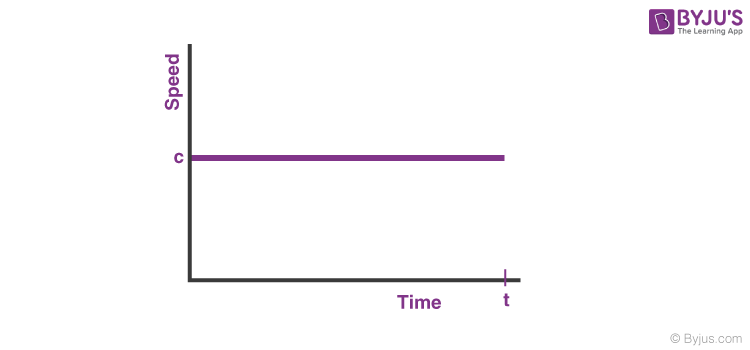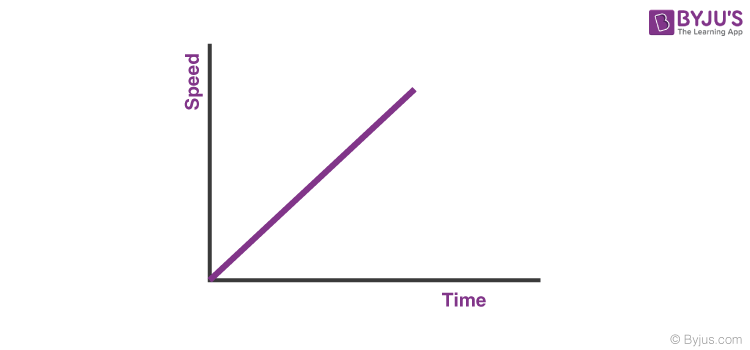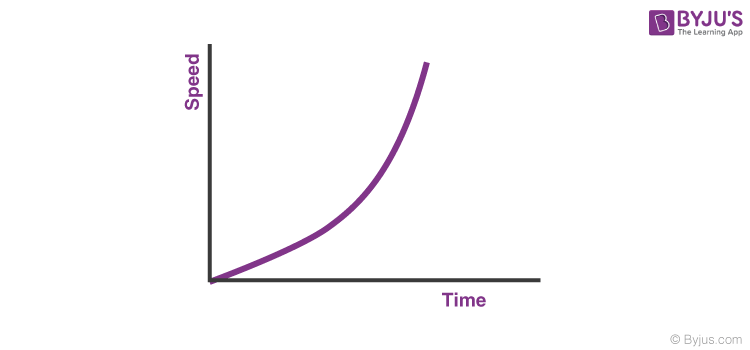# Visualization of Speed - Time Graphs

We have learnt about what speed and velocity are and the difference between the two. Now, we will learn about speed-time graphs and velocity-time graphs.

## Speed – Time Graphs

We know that the speed at a certain time is the magnitude of the velocity at that time. So we can follow equations of motion to draw speed time graphs.

### Case 1: Speed-time graphs with constant speed (zero acceleration)

When the speed is constant, the speed time graph, with Y-axis denoting speed and the X-axis denoting time, will be like this:Time graph for constant speed

Speed-

As clear from the graph, the speed is constant (c) throughout the time interval. No matter how much the time changes, the speed will be c at every instant.

Example: If the acceleration of a particle is zero (0), and speed is constant, say 5 m/s at t =0, then it will remain constant throughout the time.

### Case 2: Speed-time graphs with constant acceleration

When the acceleration is constant, and the initial speed of the particle is zero, the speed of the particle will increase linearly, as predicted by the equation:

v = u + at

Since u = 0

v = atSpeed-Time graph with constant acceleration

As shown in the figure, the speed of the particle will increase linearly with respect to time. The slope of the graph will give the magnitude of acceleration.

Example: If the acceleration of a particle is constant (k) and the initial speed is zero, the speed increases or decreases linearly. The slope of the speed-time graph will give the magnitude of acceleration (k).

### Case 3: Speed-time graphs with increasing acceleration

When the acceleration increases with time, the speed time graph will be a curve, as predicted from the equation:

v = u + at

Since u = 0

v= at

Since acceleration is a function of time, the speed time graph will be a curve.

Note: Since the acceleration is continuously increasing with time, the magnitude of the slope will also continuously increase with time.Speed-time graph with increasing acceleration

Example: If the acceleration of a particle is a function of time and the initial speed is zero, the speed-time graph will be a curve. The slope of the speed-time graph at any instant (at a certain time) will give the magnitude of acceleration at that time. Case 3 is just the case of increasing acceleration. There will be a different graph when the acceleration decreases with time.

## Frequently Asked Questions – FAQs

Q1

### What is velocity?

The speed of a body in a specific direction is the measure of Velocity. Rate of change in displacement with respect to time is known as velocity. Velocity is a vector quantity that has both magnitude and direction.
Q2

### What is speed?

Speed is measured as the ratio of distance to the time in which the distance was covered. Speed is a scalar quantity as it has only direction and no magnitude.
Q3

### How many types of speed are there?

There are four types of speed and they are:
• Uniform speed
• Variable speed
• Average speed
• Instantaneous speed
Q4

### What is instantaneous speed?

When an object is moving with variable speed, then the speed of that object at any instant of time is known as instantaneous speed.
Q5

### Define average velocity.

Average velocity is a vector quantity. Average velocity is defined as the change in position or displacement (∆x) divided by the time intervals (∆t) in which the displacement occurs.The average velocity can be positive or negative depending upon the sign of the displacement. The SI unit of average velocity is metres per second (m/s or ms-1).

#### Complete quiz of the chapter Motion Class 9 Science#### Top 10 NTSE Important Questions on Motion Class 9Test Your Knowledge On Speed Time Graphs!